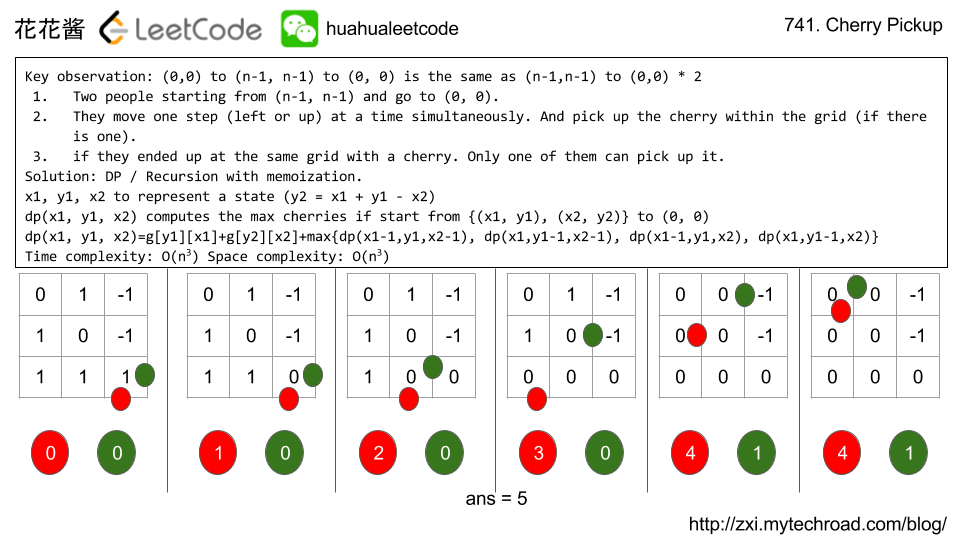Problem:

In a N x N grid representing a field of cherries, each cell is one of three possible integers.

• 0 means the cell is empty, so you can pass through;
• 1 means the cell contains a cherry, that you can pick up and pass through;
• -1 means the cell contains a thorn that blocks your way.

Your task is to collect maximum number of cherries possible by following the rules below:

• Starting at the position (0, 0) and reaching (N-1, N-1) by moving right or down through valid path cells (cells with value 0 or 1);
• After reaching (N-1, N-1), returning to (0, 0) by moving left or up through valid path cells;
• When passing through a path cell containing a cherry, you pick it up and the cell becomes an empty cell (0);
• If there is no valid path between (0, 0) and (N-1, N-1), then no cherries can be collected.

Example 1:

Note:

• grid is an N by N 2D array, with 1 <= N <= 50.
• Each grid[i][j] is an integer in the set {-1, 0, 1}.
• It is guaranteed that grid and grid[N-1][N-1] are not -1.

Idea:

DP

Key observation: (0,0) to (n-1, n-1) to (0, 0) is the same as (n-1,n-1) to (0,0) twice

1. Two people starting from (n-1, n-1) and go to (0, 0).
2. They move one step (left or up) at a time simultaneously. And pick up the cherry within the grid (if there is one).
3. if they ended up at the same grid with a cherry. Only one of them can pick up it.

Solution: DP / Recursion with memoization.

x1, y1, x2 to represent a state y2 can be computed: y2 = x1 + y1 – x2

dp(x1, y1, x2) computes the max cherries if start from {(x1, y1), (x2, y2)} to (0, 0), which is a recursive function.

Since two people move independently, there are 4 subproblems: (left, left), (left, up), (up, left), (left, up). Finally, we have:

dp(x1, y1, x2)= g[y1][x1] + g[y2][x2] + max{dp(x1-1,y1,x2-1), dp(x1,y1-1,x2-1), dp(x1-1,y1,x2), dp(x1,y1-1,x2)}

Time complexity: O(n^3)

Space complexity: O(n^3)# Solution: DP

Time complexity: O(n^3)

Space complexity: O(n^3)

## Java

Related Problems:

If you like my articles / videos, donations are welcome.

Buy anything from Amazon to support our website## Be First to Comment

Mission News Theme by Compete Themes.